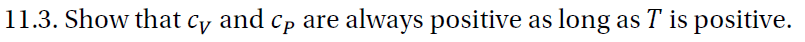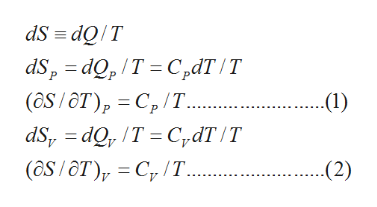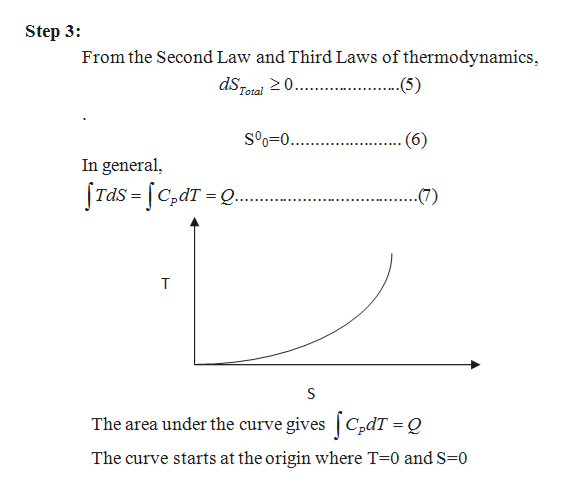# 11.3. Show that cy and cp arealways positive as long as T is positive.

Question
16 viewshelp_outlineImage Transcriptionclose11.3. Show that cy and cp are always positive as long as T is positive. fullscreen
check_circle

Step 1

Heat capacities are defined in terms of quantity of heat and are related to entropy changes.

The relations connecting heat capacities to the quantity of heat Q and the entropy changes (dS) are given as follows:help_outlineImage TranscriptionclosedS = dQ/T dS, = dQ, /T = C„dT /T (ôS / ôT), = C, /T.. dS, = dQ, /T = C,dT /T (ôS / ôT), = C, /T... P. ..(1) .(2) fullscreen
Step 2

Equation (1) shows that CP is related to the isobaric change in entropy

Equation (2) shows that CV is related to the isochoric change in entropy

From equations (1) & (2), we can write:

Step 3

From the Second Law and Third ...help_outlineImage TranscriptioncloseStep 3: From the Second Law and Third Laws of thermodynamics, dSee 20. . ..(5) Total S°o=0.. . (6) In general, [Tds = [C,dT = Q. (7) The area under the curve gives | C,dT = Q The curve starts at the origin where T=0 andS=0 fullscreen

### Want to see the full answer?

See Solution

#### Want to see this answer and more?

Solutions are written by subject experts who are available 24/7. Questions are typically answered within 1 hour.*

See Solution
*Response times may vary by subject and question.
Tagged in

### Chemical Engineering